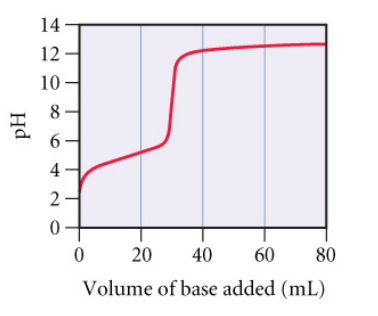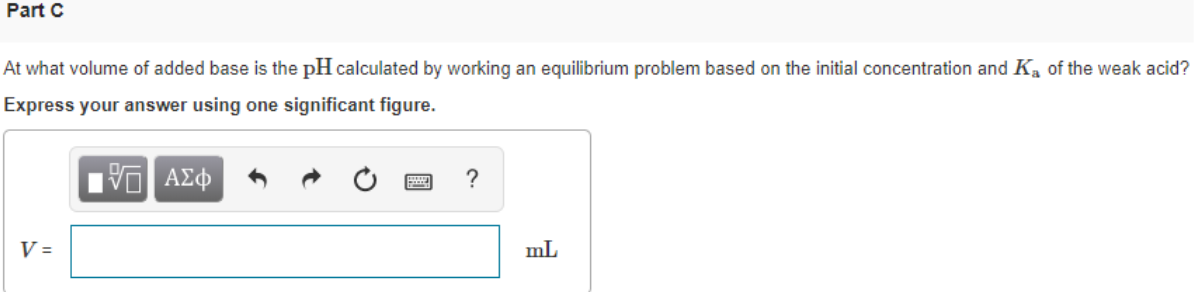# Consider the following curve (Figure 1) for the titration of a weak monoprotic acid with a strong base and answer each of the following questions. At what volume of added base is the pH calculated by working an equilibrium problem based on the initial concentration and Ka of the weak acid? Express your answer using one significant figure.